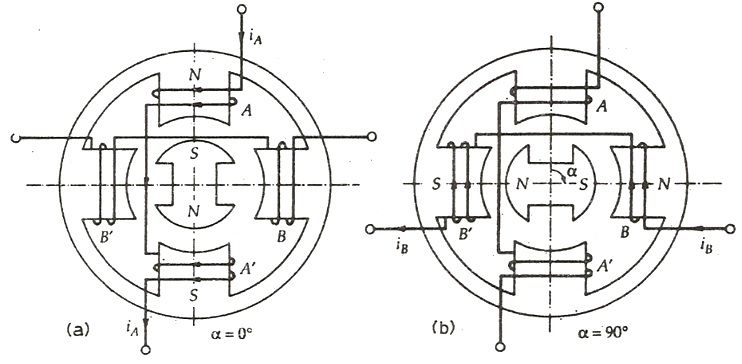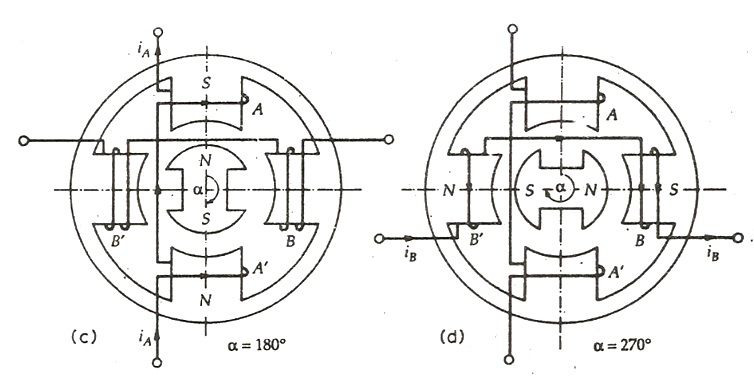You are here: / / / PM Stepper Motor

# PM Stepper Motor

Views:24     Author:Site Editor     Publish Time: 2018-01-15      Origin:Site

The Permanent Magnet Stepper Motor has a stator construction similar to that of the single stack variable reluctance motor. The rotor consists of permanent magnet poles of high retentivity steel and is cylindrical in shape. The concentrating windings on diametrically opposite poles are connected in series to form a two phase winding on the stator.

The rotor poles align with the stator teeth depending on the excitation of the winding. The two coils AA’ connected in series to form a winding of Phase A. Similarly the two coil BB’ is connected in series forming a phase B windings.The figure below shows 4/2 Pole Permanent Magnet Stepper Motor.In figure (a) the current flows start to the end of phase A. The phase winding is denoted by Aand the current by i+A. The figure shows the condition when the phase winding is excited with the current i+A. The south pole of the rotor is attracted by the stator phase A. Thus, the magnetic axis of the stator and rotor coincide and α = 0⁰

Similarly, in the figure (b) the current flows from the start to the end at phase B. The current is denoted by i+B  and the winding by B+. Considering the figure (b), the windings of phase A does not carry any current and the phase B is excited by the i+B   current. The stator pole attracts the rotor pole and the rotor moves by 90⁰ in the clockwise direction. Here α = 90⁰

The figure (c) below shows that the current flows from the end to the start of the phase A. This current is denoted by i–A and the winding is denoted by A. The current i–A is opposite to the current i+A. Here, phase B winding is de-energized and phase A winding is excited by the current i–A. The rotor moves further 90⁰ in clockwise direction and the α = 180⁰In the above figure (d), the current flows from end to starting point of phase B. The current is represented by i–B and the winding by B. Phase A carries no current and the phase B is excited. The rotor again moves further 90⁰ and the value of α = 270⁰

Completing the one revolution of the rotor for making α = 360⁰ the rotor moves further 90 degrees by de-energizing the winding of phase B and exciting the phase A. In the permanent magnet stepper motor the direction of the rotation depends on the polarity of the phase current.The sequence A+, B+, A, B, A+ is followed by the clockwise movement of the rotor and for the anticlockwise movement, the sequence becomes A+ B, A, B+, A+.

The permanent magnet rotor with large number of poles is difficult to make, therefore, stepper motors of this type are restricted to large step size in the range of 30 to 90⁰. They have higher inertia and therefore, lower acceleration than variable stepper motors.